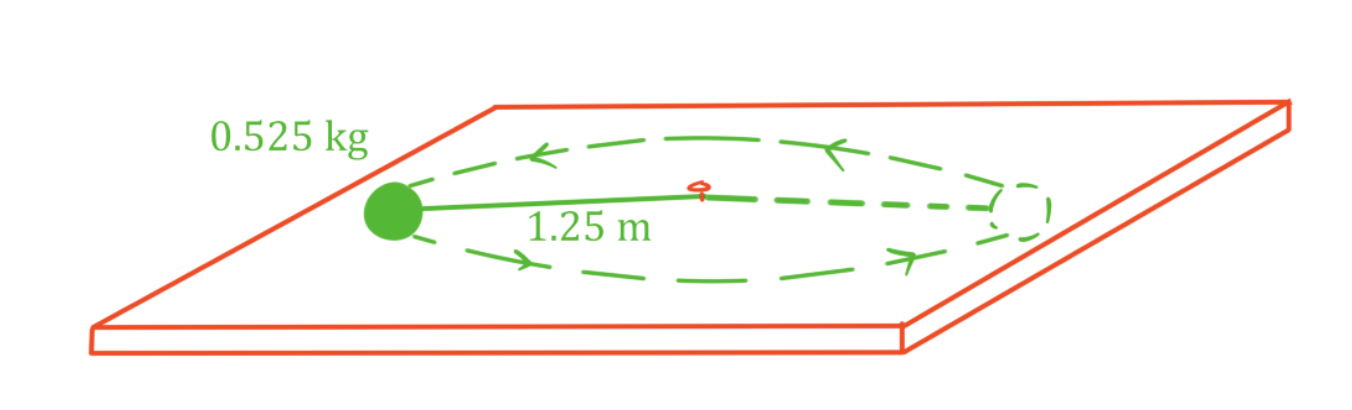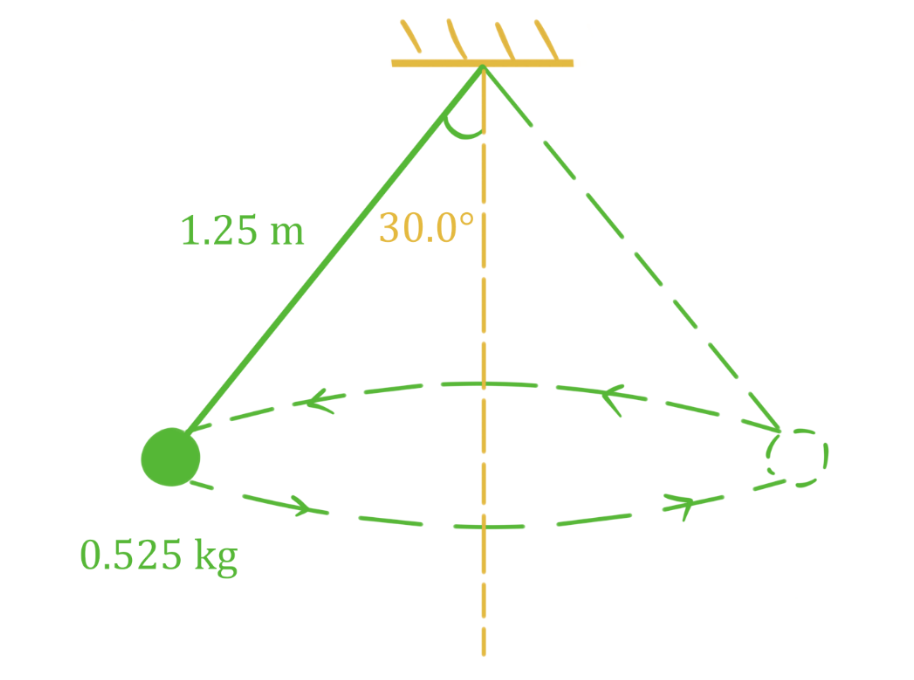# Horizontal circular motion

### Horizontal circular motion

#### Lessons

In this lesson, we will learn:
• Meaning of uniform circular motion
• Solving problems involving horizontal circular motion

Notes:

• An object moving in a circular path is in circular motion. If the speed of the object is constant, it is uniform circular motion.
• An object in uniform circular motion does experience acceleration, even though its speed is constant. Remember, acceleration is change in velocity, and a velocity is made up of speed and direction. For the object to move in a circle, the direction of its velocity must change constantly. This change in direction is the acceleration, called centripetal acceleration ("centripetal" means "towards the center"). For an object moving in a circular path, the centripetal acceleration vector is always pointed towards the center of the circle.
• Like any other type of acceleration, centripetal acceleration is caused by a force (called centripetal force). The centripetal force vector also always points towards the center of the circle.
• In order for an object to be moving in a circular path, the net force acting on the object must be a centripetal force (a force that always is pointed towards the center). When multiple forces act on an object in circular motion, those forces must add up to a centripetal force. It is important to understand that centripetal force is not a separate force that acts on an object. It is a net force which follows a specific rule: it always points towards the center of the circular path.
• In a horizontal circular motion problem, any forces acting on the object in the vertical direction must balance so that $\Sigma F_{vertical} = 0N$ (otherwise the object would accelerate vertically). Only horizontal forces will contribute to the net force causing circular motion.
Period and Frequency

$T = \frac{total time}{\# of revolutions}$

$f = \frac{\# of revolutions}{total time}$

$T = \frac{1}{f}$

$T:$ period, in seconds (s)

$f:$ frequency, in hertz (Hz)

Centripetal Acceleration

$a_{c} = \frac{v^{2}}{r} = \frac{4\pi^{2}r}{T^{2}}$

$a_{c}:$ centripetal acceleration, in meters per second squared $(m/s^{2})$

$v:$ velocity, in meters per second (m/s)

$r:$ radius, in meters (m)

$T:$ period, in seconds (s)

• 1.
Ball on horizontal string moving in circular motion

A 0.525 kg ball is attached to a 1.25 m string and swings in a circular path, making 2.00 revolutions per second. The angle of the string is nearly horizontal. Find the tension in the string.• 2.
Ball on angled string in horizontal circular motion

A 0.525 kg ball is attached to a 1.25 m string and swings in a circular path. The angle of the string away from vertical is 30.0°. Find the centripetal force acting on the ball and the speed of the ball.